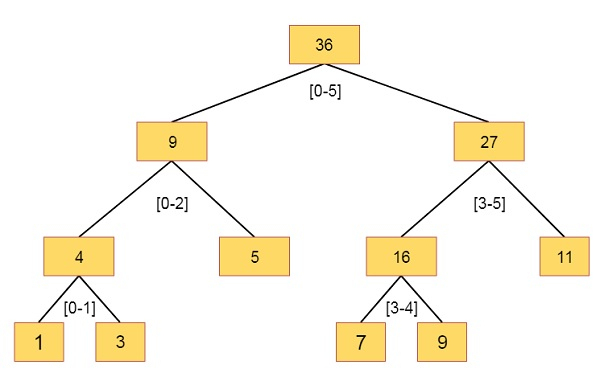# Segment Trees in Data Structure

In this section we will see what is the segment tree. Before discussing that, let us see one problem.

Suppose we have an array arr[0,…,n-1], We can do following operations −

• Find the sum of elements from index l to r, where 0 ≤ l ≤ r ≤ n-1

• Change the value of a specified element of the array to a new value x. We need to do arr[i] = x. The i in range 0 to n – 1.

We can solve this problem by using the Segment tree. The segment tree can help us to get the sum and query in O(log n) time. So let us see how to represent this −

• Leaf nodes are the elements of the given array

• Each internal nodes are representing some merging of leaf nodes. The merging may be different in different cases. Here merging is the sum of leaves under a node.

Suppose we have an array like [1, 3, 5, 7, 9, 11]. So the segment tree will be